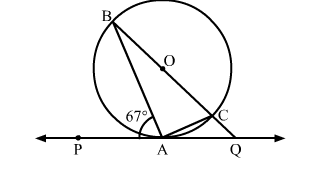# In the given figure, PQ is a tangent to a circle with centre O.`
Question:

In the given figure, PQ is a tangent to a circle with centre O. A is the point of contact. If  ∠PAB =  67, then the measure of ∠AQB is
(a) 73
(b) 64
(c) 53
(d) 44Solution:

We know that a chord passing through the centre is the diameter of the circle.
∵∠BAC = 90    (Angle in a semi circle is 90)
By using alternate segment theorem
We have ∠PAB = ∠ACB = 67
Now, In △ABC
∠ABC + ∠ACB + ∠BAC = 180            [Angle sum property of a triangle]
⇒ ∠ABC + 67∘ +  90 = 180
⇒ ∠ABC= 23
Now, ∠BAQ = 180 − ∠PAB          [Linear pair angles]
= 180 − 67
= 113
Now, In △ABQ
∠ABQ + ∠AQB + ∠BAQ = 180            [Angle sum property of a triangle]
⇒ 23 + ∠AQB + 113 = 180
⇒ ∠AQB = 44
Hence, the correct answer is option (d).